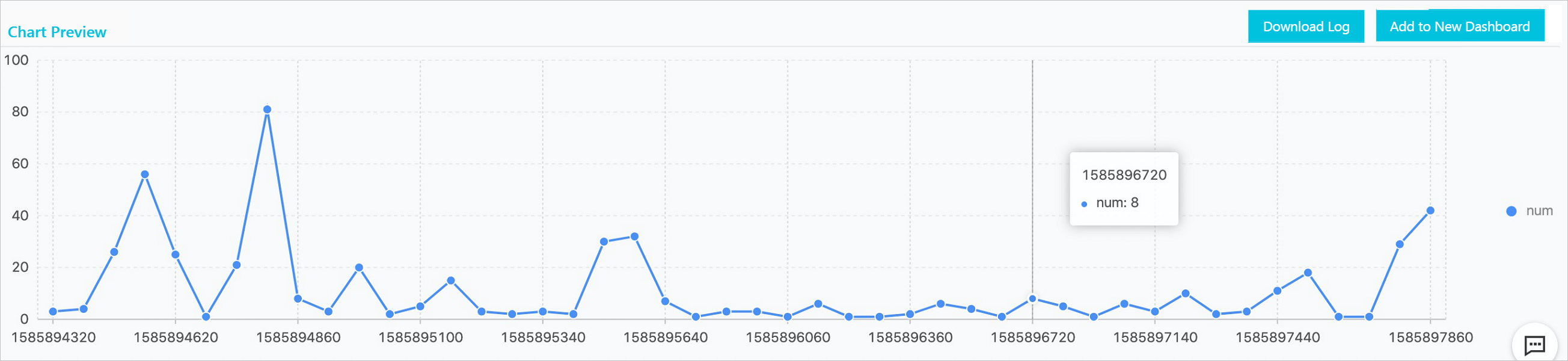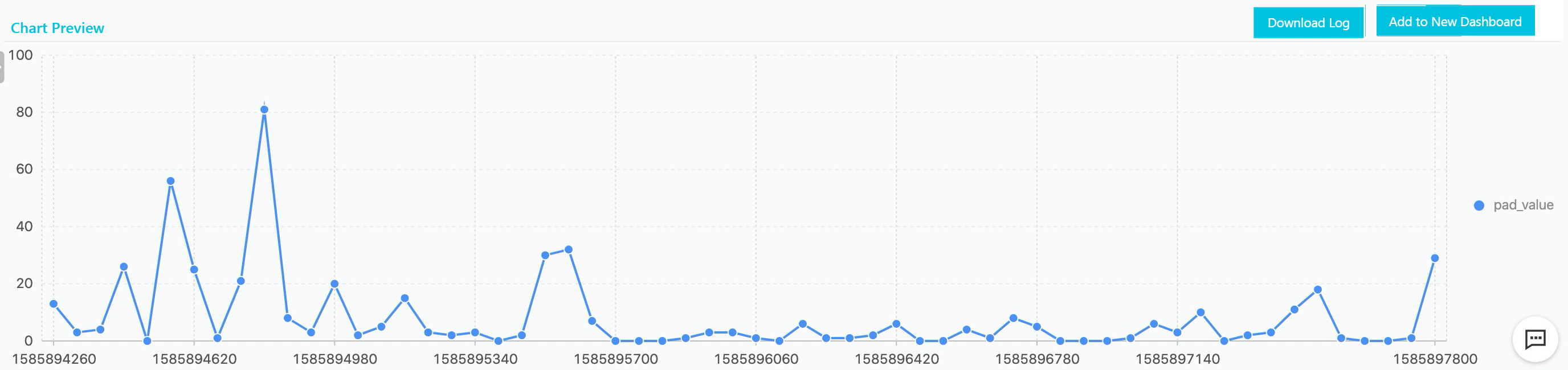The time series padding function pads data points that are missing in a time series.

• Function expressions
``select series_padding(long stamp, double value, long interval, varchar padType)``
• Input parameters
Parameter Description
stamp The UNIX timestamp of the data.
value The numeric data that corresponds to each specified point in time.
interval The interval at which data is collected. For example, if data is collected every 10 seconds, the interval is 10.
padType The type of padded data points. Valid values: zero, mean, forward, and backward.
• mean: pads the average of the valid values on both sides of a missing data point.
• forward: pads the valid value on the left of a missing point.
• backward: pads the valid value on the right of a missing point.
• Result
``````unixtime   |       pad_value
-------------+-----------------------
1.5513696E9 |   0.11243584740434608
1.5513732E9 |   0.09883780706698506
1.5513768E9 |   0.08240823914341992
1.5513804E9 |    0.0728240514818139
1.551384E9 |   0.05888517541914705
1.5513876E9 |   0.04953931499029833
1.5513912E9 |  0.043698605551761895
1.5513948E9 |   0.04400292632222124
1.5513984E9 |   0.04727081764249449
1.551402E9 |  0.054632234293121314
1.5514056E9 |   0.05331214064978596
1.5514092E9 |   0.05093117289934144
1.5514128E9 |  0.053620170319174806
1.5514164E9 |   0.05405914786225842``````
• Examples
Execute the following query statement and select the line chart to display the query results. Several data points are missing on the line chart, as shown in the following figure.
``* and Method: GetLogStoreLogs and ProjectName: lunar and LogStore: geos and Latency > 800000 | select __time__ - __time__ 60% as time, COUNT(*) * 1.0 as num from log group by time order by time asc limit 1000``Execute the following query statement that includes the time series padding function, and select the line chart to display the query results. The missing data points are padded, as shown in the following figure.
``* and Method: GetLogStoreLogs and ProjectName: lunar and LogStore: geos and Latency > 800000 | select series_padding(time, num, 60, 'zero') from (select __time__ - __time__ 60% as time, COUNT(*) * 1.0 as num from log group by time order by time asc limit 1000)``